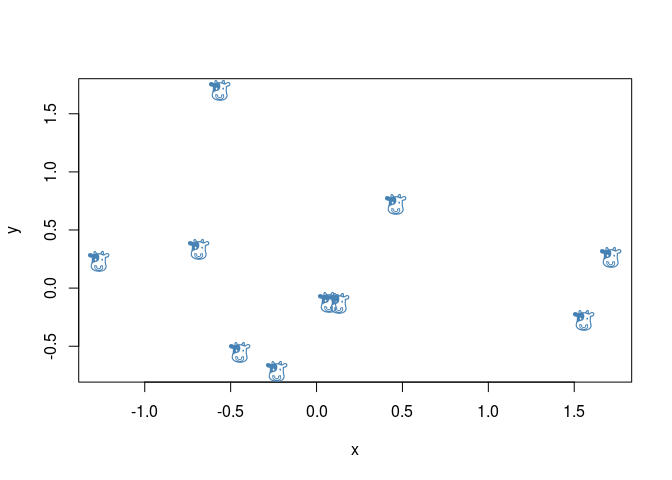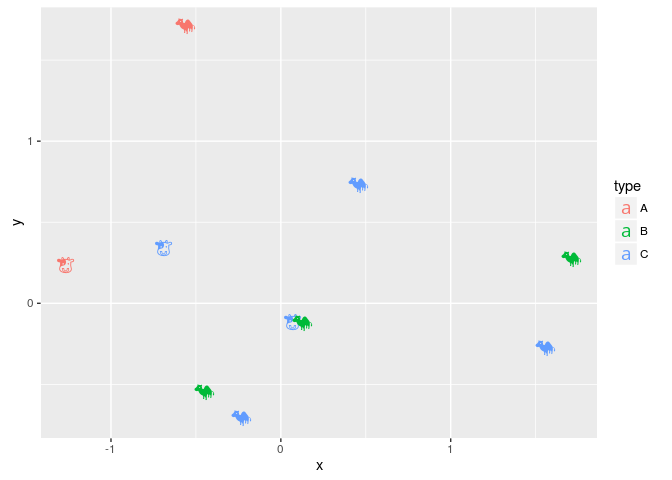![](http://guangchuangyu.github.io/blog_images/2015/Screenshot 2015-12-16 10.55.49.png)

I have played with emoji in `R` for a while. My solution of using it is different from what implemented in emoGG.

`emoGG` is a good attemp to add `emoji` in `ggplot2`. It render `emoji` picture (png) and creat a layer, `geom_emoji`, to add emoji.

In my opinion, `emoji` should be treated as ordinary font in user interface, albeit it maynot be true internally.

It would be more flexible if we can use emoji as ordinary font and in this way user don’t need to learn extra stuff.

I implemented my solution of using `emoji` in the R package emojifont. The package is very simple, pack some emoji fonts (currently only OpenSansEmoji.ttf) and use showtext to render the fonts, then we can use the font in either base plot or ggplot2.

# emojifont

## Installation

``````devtools::install_github("GuangchuangYu/emojifont")
``````

``````library(emojifont)
## list available emoji fonts
list.emojifonts()

##  "OpenSansEmoji.ttf"

``````

# Emoji characters

To use `emoji`, we need to use their corresponding unicode. Emoji unicode can be found in http://apps.timwhitlock.info/emoji/tables/unicode, or searched using remoji package.

# Emoji in R plot

## base plot

``````set.seed(123)
x <- rnorm(10)
set.seed(321)
y <- rnorm(10)
plot(x, y, cex=0)
text(x, y, labels=emoji('cow'), cex=1.5, col='steelblue', family='OpenSansEmoji')
``````## ggplot2

``````d <- data.frame(x=x, y=y,
label = sample(c(emoji('cow'), emoji('camel')), 10, replace=TRUE),
type = sample(LETTERS[1:3], 10, replace=TRUE))
require(ggplot2)
ggplot(d, aes(x, y, color=type, label=label)) +
geom_text(family="OpenSansEmoji", size=5)
``````We can also use `emoji` in title, legend or axis label.

``````dd=data.frame(x=emoji(c("satisfied", "disapointed")), y=c(50, 10))
emoji_text=element_text(family="OpenSansEmoji", size=20)
ggplot(dd, aes(x, y)) + geom_bar(stat='identity', aes(fill=x)) +
ggtitle(paste(emoji(c("+1", "-1")), collapse=" "))+
theme(axis.text.x = emoji_text, legend.text=emoji_text, title=emoji_text) +
xlab(NULL)+ylab(NULL)
``````

![](http://guangchuangyu.github.io/blog_images/2015/Screenshot 2015-12-16 11.10.51.png)

## ggtree

``````require(ggtree)
require(colorspace)

tree_text=paste0(
"(","(","(",
"(",
"(",
emoji("cow"), ",",
"(",
emoji("whale"),",",
emoji("dolphin"),
")",
"),",
"(",
emoji('pig2'),",",
emoji('boar'),
")",
"),",
emoji("camel"),
"),", emoji("fish"), "),",
emoji("seedling"), ");")

geom_tiplab(family="OpenSansEmoji", size=10,
color=rainbow_hcl(8))
``````# Apple Color Emoji

Although `R`’s graphical devices don’t support `AppleColorEmoji` font, it’s still possible to use it. We can export the `plot` to `svg` file and render it in `Safari`.

``````library(gridSVG)
p <-  ggtree(read.tree(text=tree_text), size=2) + geom_tiplab(size=20)
p <- p %>% phylopic("79ad5f09-cf21-4c89-8e7d-0c82a00ce728", color="firebrick", alpha = .3)
p <- p + xlim(NA, 7) + ylim(NA, 8.5)
p